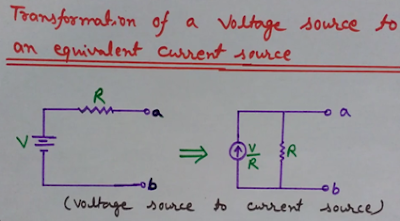## Source Transformation

Here we will understand the concept of source transformation (source conversion).
You will learn here how it is possible to convert a voltage source to a current source and a current source to a voltage source.
While analyzing the circuits, it is easy and preferable to have same type of source - either voltage source only or current source only.

## Watch the Complete Video on Source Transformation Here

For transformation, each voltage source should have a series internal resistance (impedance) and each current source a parallel internal resistance (impedance).
Here we will understand the concept of transformation of a voltage source to an equivalent current source and transformation of a current source to an equivalent voltage source.

## Transformation of a voltage source to an equivalent current source-

The diagram shown below explains the voltage source to current source conversion.Voltage Source to Current Source Conversion

As we know that for transformation each voltage source should have a series internal resistance while a current source should have a parallel internal resistance.
As you can see in the diagram shown above, that this voltage source (V) is having an internal resistance (R) in series with it. So we can change this voltage source into its equivalent current source.
From the diagram you can see that to obtain current source from this voltage source we need to divide the value of voltage source by its internal resistance (V/R) and put this resistance (R) in parallel to this current source (V/R).
Here observe that the direction of current source is same as that of the voltage source.
So in this way we can convert a voltage source into its equivalent current source.
Now we will discuss how to convert a current source into its equivalent voltage source

## Transformation of a current source to an equivalent voltage source

The diagram given below will help you in understanding the concept of converting a current source into its equivalent voltage source.Current Source to Voltage Source Conversion

In the left part of this diagram you can see that, there is a resistance (R) in parallel to a current source (I). Consider it as its internal resistance.
Now to convert this current source into voltage source, simply multiply the values of the current source and its internal resistance (IR) and place this resistance (R) in series with this current source. This transformation is clear by the diagram give below.
In this case also the direction of new voltage source is same as that of the current source.

So in this way we can inter-convert voltage and current sources easily as per our requirements.

FREQUENCY SPECTRUM OF AMPLITUDE MODULATION (WAVEFORMS AND EQUATIONS DERIVATION)

AMPLITUDE MODULATION (TIME DOMAIN EQUATIONS AND WAVEFORMS)

STEP INDEX OPTICAL FIBER (MULTIMODE AND SINGLE MODE STEP INDEX FIBERS)

PULSE MODULATION TECHNIQUES (PAM, PWM, PPM, PCM)

OPTICAL FIBER: STRUCTURE AND WORKING PRINCIPLE

PULSE AMPLITUDE MODULATION (PAM)

COMPARISON OF PAM, PWM, PPM MODULATION TECHNIQUES

PULSE WIDTH MODULATION (PWM)

CONTINUOUS TIME AND DISCRETE TIME SIGNALS (C.T. AND D.T. SIGNALS)

NEED AND BENEFITS OF MODULATION

PULSE POSITION MODULATION (PPM)

OPTICAL FIBERS IN COMMUNICATION: COVERS ALL IMPORTANT POINTS

OPTICAL FIBER SOURCES (DESIRABLE PROPERTIES)

SAMPLING THEOREM AND RECONSTRUCTION (SAMPLING AND QUANTIZATION)

SUPERPOSITION THEOREM (BASICS, SOLVED PROBLEMS, APPLICATIONS AND LIMITATIONS)

Digital Modulation Techniques (ASK, FSK, PSK, BPSK)/ Amplitude, Frequency and Phase Shift Keying

Conventional AM Vs DSB-SC Vs SSB-SC Vs VSB (Comparison of AM Systems)

What are Microwaves and their Applications (Uses) in various fields

Basic Structure of Bipolar Junction Transistor (BJT) - BJT Transistor - Working and Properties

Polar Plots of Transfer Functions in Control Systems (How to Draw Nyquist Plot Examples)

Generation of Binary Phase Shift Keying (BPSK Generation) - Block Diagram of Binary Phase Shift Keying (BPSK)

Low Level and High Level Modulation Block Diagram (AM Transmitter Block Diagram)

Block Diagram of CRO (Cathode Ray Oscilloscope), Components of CRO and CRT with Structure and Working

Slope Overload Distortion and Granular (Idle Noise), Quantization Noise in Delta Modulation

Frequency Translation/Frequency Mixing/Frequency Conversion/Heterodyning (Basic Concepts and Need)

Quadrature Phase Shift Keying Modulation (QPSK) Basics, Waveform and Benefits

Pulse Code Modulation (PCM) Vs Differential Pulse Code Modulation (DPCM)